About   Products   Support   Videos   Purchase   Contact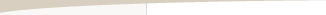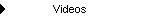Patience is a Necessity

by Michael R. Bryant

Patience may be a virtue, but when it comes to trading, patience is a necessity. What do I mean by that? Let's say you've developed a good trading strategy. I'm not going to discuss in this article what makes it a good strategy or even how you know it's good. Let's just assume it's a good strategy, by which I mean it's inherently profitable. How long do you need to trade it before you're profitable? How many trades will you need to make before you can reasonably expect to show a profit? Or, to put it another way, how long can you trade it without a profit before you know something is wrong? That's the topic of this article, and the short answer is "longer than you probably think."

Given the challenges of developing a profitable trading strategy, it's easy to become focused solely on that task. Then when you finally start trading your strategy, you suddenly realize you're not sure how it's going. Is it ok to be down after 10 trades, flat after 20, going nowhere fast after 30? The historical sequence showed a profit after a few trades. Sure, there will be drawdowns, but how long should you keep going before throwing in the towel and starting over?

The Historical Sequence and Monte Carlo Analysis

The historical sequence of trades from a trading strategy is simply the set of trades listed in the order in which they occurred during back-testing (historical testing) of the strategy. Most traders rely on the performance results from the historical sequence of trades to guide their expectations when starting to trade a new strategy. However, metrics such as the maximum number of consecutive losses, longest flat period, worst-case drawdown, and others, suffer from sampling bias. This means the value of those metrics depends on the specific trades that were analyzed and the order in which they occurred. If a different set of trades from the same strategy were analyzed, different values would more than likely be calculated for the same metrics.

Monte Carlo analysis is one way to deal with this sampling problem. From a statistical point of view, the historical sequence of trades is just one specific sequence of trades sampled from the probability distribution of trades for a given strategy. Monte Carlo analysis provides a way to approximate the probability distribution of trades using only the historical sequence.

It does this by repeatedly scrambling the order of the trades in the historical sequence. Each scrambled sequence is analyzed just like the historical sequence, and the results for each sequence combine to build up the approximate distribution. Probability results can then be determined directly from the results for all sequences. For example, if 1000 trade sequences were analyzed, and in 950 of them, the worst-case drawdown was less than 23%, then you could say that the probability of having a worst-case drawdown less than 23% was 95% (950/1000).

Using Monte Carlo Analysis to Answer "How Long?"

Market System Analyzer (MSA) includes a Monte Carlo analysis feature that works as described above. Typical results generated by this feature are shown below. For example, the first table shows that as the required confidence level increases, the rate of return declines and the maximum drawdown increases. For example, the return is 47.5% at a confidence level of 90% but only 42.4% at a confidence level of 99%. The second table shows some typical results at a particular confidence level; in this case, 95%. For example, the average winning trade is \$752 with 95% confidence. This means that in 95% of the Monte Carlo samples, the average winning trade was at least \$752.

 Confidence (%) Return (%) Max Drawdown (%) 80 49.31 7.539 85 48.54 7.974 90 47.51 8.58 91 47.27 8.729 92 47.01 8.897 93 46.68 9.092 94 46.37 9.321 95 45.97 9.598 96 45.59 9.892 97 45 10.21 98 43.98 10.73 99 42.43 11.67 100 33.14 17.05

 Monte Carlo Results at 95.00% Confidence Total Net Profit: \$45,970.00 Max Number of Contracts: 2 Final Account Equity: \$145,970.00 Minimum Number of Contracts: 1 Return on Starting Equity: 45.97% Average Number of Contracts: 1 Profit Factor: 1.463 Largest Winning Trade: \$2,880.00 Largest Losing Trade: (\$1,880.00) Largest Winning Trade (%): 2.151% Largest Losing Trade (%): -1.470% Average Winning Trade: \$751.80 Average Losing Trade: (\$765.73) Average Winning Trade (%): 0.6351% Average Losing Trade (%): -0.5845%

In addition to these results, the Monte Carlo analysis in MSA has an optional result that can be used to help address the question of how long it takes to be profitable. The option "Probability that account equity will be X% higher after N trades" is available on the Analysis Setup screen on the Options tab. There's a companion option for "Probability that account equity will be X% lower after N trades."

As an example, if you enter 50 for X and 30 for N, the Monte Carlo results will include "Probability that account equity will be 50% higher after 30 trades". To calculate the result, the program randomly draws trades from the distribution of trades (i.e., the set of all trades in the historical sequence) and applies the settings in the program to simulate trading over the first 30 trades that it selects. It then repeats this process for each Monte Carlo sample. Finally, it calculates the percentage of samples for which the account equity was higher by 50% at the end of the 30 trade period.

To answer the question of how long it takes to be profitable, a value of 0 can be entered for X, and different values of N can be tried until one is found such that the confidence level reaches a pre-determined level, such as 95%, which is a typical level used for statistical testing. In other words, we want to ask how many trades it takes for the profit to be at least zero (breakeven) with 95% confidence. In the results that follow, it typically took only a few iterations on N to find the number of trades that resulted in 95% confidence.

How Long Did it Take?

Three example strategies were analyzed to illustrate how long it can take to be profitable. The three strategies are ones discussed in recent newsletter articles:

1. MiniMax Clone Strategy from "Reverse Engineering a Trading Strategy"

3. PricePatterns Strategy from "Price Pattern Strategies"

In each case, the Monte Carlo option discussed above was used to find the number of trades such that the strategy was at least breakeven with 95% confidence. Since each of the three strategies trades the futures market, the analysis was performed first with one contract per trade. Fixed fractional trading was then applied, and the number of trades for breakeven trading at 95% confidence was calculated for different values of the fixed fraction. For fixed fractional trading, a starting account size of \$100,000 was used in each case.

MiniMax Clone Strategy

This strategy trades the E-mini S&P 500 futures (ES.D) on daily bars with an average holding time of about one week. Based on the one-contract equity curve, shown below in Fig. 1, it appears that the strategy should be profitable fairly quickly.Figure 1. One-contract equity curve for the MiniMax Clone strategy on daily bars of the E-mini S&P 500 futures.

However, when the Monte Carlo analysis is performed, it's found that it takes 54 trades before the strategy is profitable with 95% confidence. Notice that at trade 50 in Fig. 1, the strategy is clearly profitable, which suggests that the historical sequence may not be a good guide for estimating the time to profitability.

 Download the MSA files for the three strategies used in this article.* The results with fixed fractional position sizing are shown below in Fig. 2. Notice that as the trading becomes more aggressive with higher fixed fractions,  it takes longer before the 95% confidence level is reached. With a fixed fraction of 10%, it takes 78 trades before the strategy is profitable with 95% confidence. In terms of calendar time, it takes from two to three years of trading before profitability is reached with 95% confidence for this strategy.Figure 2. Number of trades required for profitability at 95% confidence with fixed fractional position sizing for the MiniMax Clone strategy. The worst-case drawdown of the historical sequence is shown at right.

The IdealTrades strategy runs on 15 min bars of the E-mini Russell 2000 (TF.D) and exits each trade by the end of the day. The one-contract equity curve for this strategy is shown below in Fig. 3. With one contract per trade, the Monte Carlo analysis shows that it takes 59 trades to reach profitability at 95% confidence. This corresponds to approximately six months of trading.

If fixed fractional position sizing is applied, the number of trades increases from about 60 trades at a fixed fraction of 1% to 92 trades at a fixed fraction of 10%, as shown below in Fig. 4.Figure 3. One-contract equity curve for the IdealTrades strategy on 15 min bars of the E-mini Russell 2000 futures.Figure 4. Number of trades required for profitability at 95% confidence with fixed fractional position sizing for the IdealTrades strategy. The worst-case drawdown of the historical sequence is shown at right.

PricePatterns Strategy

The PricePatterns strategy trades the E-mini Russell 2000 on 10 min bars. Each trade is exited no later than the day's close. The one-contract equity curve is shown below in Fig. 5. The Monte Carlo results for one-contract trading show that it takes 138 trades to ensure profitability at 95% confidence. This takes about five months of trading.

If fixed fractional position sizing is applied, the number of trades increases from about 138 trades at a fixed fraction of 1% to 196 trades at a fixed fraction of 5%, as shown below in Fig. 6. At a fixed fraction of 5%, the time to reach profitability increases to about seven months.Figure 5. One-contract equity curve for the PricePatterns strategy on 10 min bars of the E-mini Russell 2000 futures.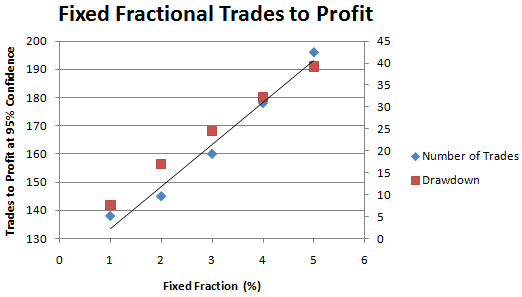Figure 6. Number of trades required for profitability at 95% confidence with fixed fractional position sizing for the PricePatterns strategy. The worst-case drawdown of the historical sequence is shown at right.

Conclusions

It's easy to imagine that trading profits will come quickly, especially if the historical testing looks promising. However, the Monte Carlo results presented above suggest that patience may be required. The MiniMax Clone strategy displays a pretty straight equity curve. However, an unfavorable sequence of trades can extend the time to profitability to two years or more, depending on position sizing. Even the day trading examples took five and six months, respectively, to ensure profits with 95% confidence.

In each example, the time to profitability at 95% confidence was longer than what one would observe just looking at the equity curve. This suggests that even if the Monte Carlo results are somewhat conservative, it's advisable not to rely on the historical results when estimating how long it will take to achieve profitability.

The flip side of looking at the probability of a return greater than zero is the probability of a return less than zero. This is provided by the other Monte Carlo option in MSA. However, if we want to know the probability of losing money after N trades, it's not necessary to repeat the calculations. The probability of losing money after N trades is 1 - P, where P is the probability of making money after N trades. For example, with the IdealTrades strategy, it takes 59 trades to make money with 95% confidence. This means there's only a 5% chance that you'll lose money after 59 trades. So if the strategy is not profitable after 59 trades (with one-contract trading), you can conclude that something is wrong.

Monte Carlo analysis can be used to provide an answer to the question of how long it might take to be profitable when starting to trade a strategy. The same analysis can also help answer the question of how long you might need to trade a strategy before concluding something is wrong. As the examples presented here illustrate, it may take longer than you expect to conclude whether or not your strategy is trading normally. In other words, when it comes to trading, patience is a necessity.

Mike Bryant

HYPOTHETICAL OR SIMULATED PERFORMANCE RESULTS HAVE CERTAIN INHERENT LIMITATIONS. UNLIKE AN ACTUAL PERFORMANCE RECORD, SIMULATED RESULTS DO NOT REPRESENT ACTUAL TRADING. ALSO, SINCE THE TRADES HAVE NOT ACTUALLY BEEN EXECUTED, THE RESULTS MAY HAVE UNDER- OR OVER-COMPENSATED FOR THE IMPACT, IF ANY, OF CERTAIN MARKET FACTORS, SUCH AS LACK OF LIQUIDITY. SIMULATED TRADING PROGRAMS IN GENERAL ARE ALSO SUBJECT TO THE FACT THAT THEY ARE DESIGNED WITH THE BENEFIT OF HINDSIGHT. NO REPRESENTATION IS BEING MADE THAT ANY ACCOUNT WILL OR IS LIKELY TO ACHIEVE PROFITS OR LOSSES SIMILAR TO THOSE SHOWN.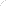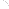Join Our Email List Email: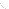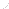For Email Marketing you can trust